Custom Search
If you want to discuss more on this or other issues related to Physics, feel free to leave a message on my Facebook page.

## Saturday, November 21, 2009

### How to determine uncertainty in a derived quantity when powers, root,etc are involved.

As we have seen in the first two part of this series it is easier to determine the uncertainty when addition and subtraction is involved but also to determine uncertainty when multiplication and division is involved.

Today we are going to see how to determine the uncertainty when a power is involved.

Very often a derived quantity is determined basic or othere derived quantities using powers, roots, etc.

For example

As we are going to see the method to determine the uncertainty in these three cases are similar and can be adapted to other derived quantities.

Example

Let us have a look at how to determine the uncertainty in volume.

A cube has length of L = 20.4+_0.2cm

Determine the uncertainty in volume.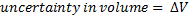V = L3

V = L*L*L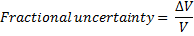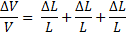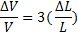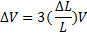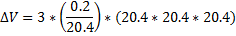=249 = 200 (1 sf because of 0.2 )

V = L3

=20.43

=8489

=8500 (2 sf because of 200)

Hence V = 8500+_200 cm3

After you have studied this example are you able to know how to find the uncertainty in any other derived quantities?

Then can you deduce the fractional uncertainty?

Can you deduce what would be the fractional uncertainty if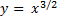?

Lastly you deduce the fractional uncertainty if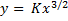?

Where K is a dimensionless constant with no uncertainty.

When there is a dimensionless constant with no uncertainty it does not enter in the equation to calculate the fractional uncertainty.

Question

The area of a circle is given by the equation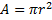If the radius r =10.1+_0.5 cm

then calculate the fractional uncertainty and the uncertainty in A.

Please not that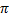is a dimentionless constant with no uncertainty and hence will play no part in thecalculations.

#### 1 comment:

1.this is actually really good